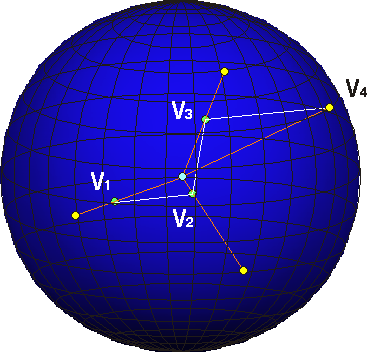Section 7The 4-D path can be normalize by finding a single 3-D space that the 4-D path is contained in. This allows a circle to be calculated from the 4-D loop in 3-D space. The center of the circle is calculated. A vector is computed from the center through each point ( V ). The longest vector is used to form a sphere. All other vectors are extended until they make contact with the surface of the sphere. Although the sphere appears 3-D, it actually exist in hyperspace ( the points are actually in different 3-D spaces ).